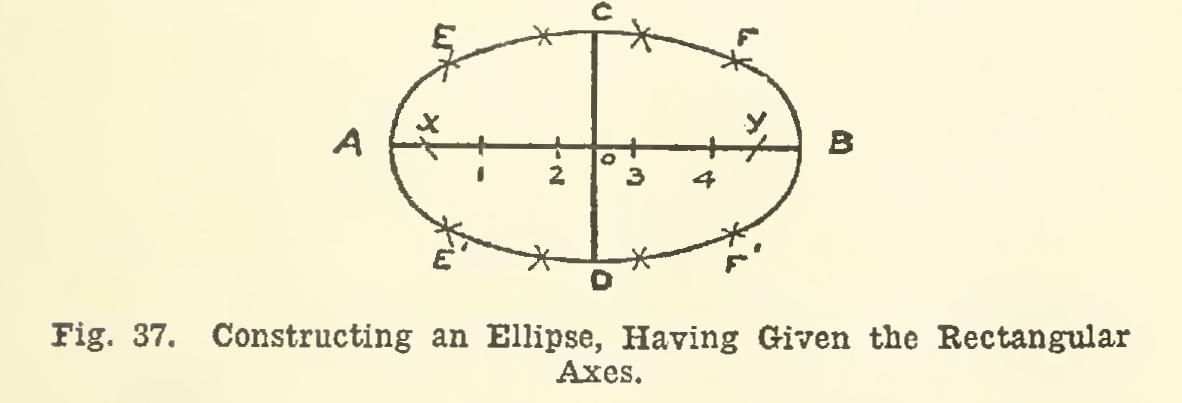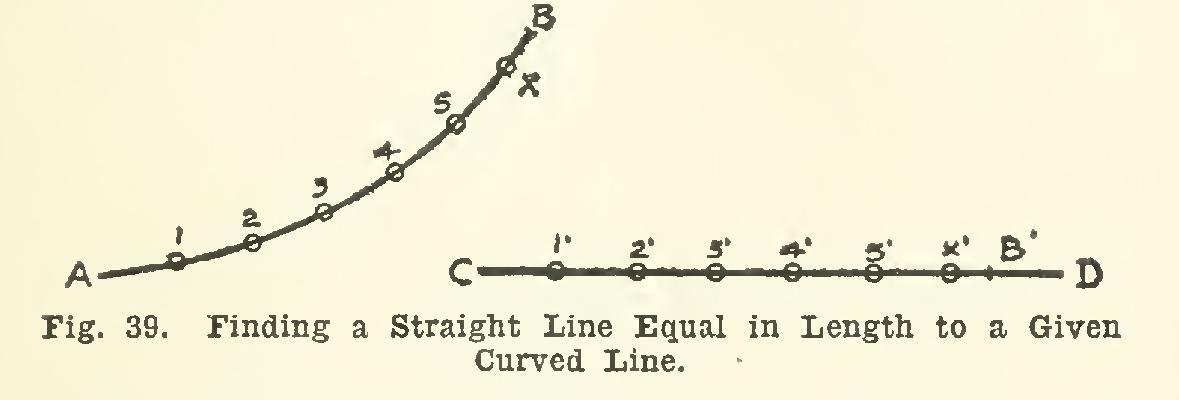Note

equal, ab, curve, length, ellipse, cd and axis

Page: 1 2

NOTE 1ŚA polygon of any number of sides which has all of its corners in the circumference of an enclosing circle, is said to be inscribed in the circle.

NOTE 2ŚThe length of the radius of a circle may be spaced around the circumference exactly six times.

To Construct an Ellipse, Having Given Two Axes at Right Angles (or the Rectangular Axes) Let AB and CD, Fig. 37, be the axes, each line bisecting the other. The point o will be the center of the ellipse. The longer axis AB is the major axis; the shorter one CD, the minor axis. With center C, and radius equal to Ao, describe an arc cutting AB in points x and y. These points are termed the foci of the ellipse.

The construction to be given depends upon the interesting property of the ellipse, that, no matter what point on the curve be taken, the sum of its distances from the foci is always the same, and is equal to the length of the major axis. Hence, to find points on the curve, mark off on AB, between x and y, any number of points, as 1, 2, 3, 4, etc. Then, with x and y as centers, and radius equal to A-1, strike four short arcs, two above and two below AB; and with the same centers, and radius equal to 1-B, describe four other arcs, cutting the first four at E, E', F, and F'. Repeat this process for the other points marked on AB, taking next A-2 as length of first radii, and 2-B as that of the second, obtaining four more points; and so on for the remaining points. The curve should be first sketched freehand through the points found, then drawn in smoothly with the irregu lar curve.To Construct an Ellipse with a Trammel, Having Given the Rectangular Axes.

In Fig. 38, AB and CD are the axes of the desired ellipse. The trammel K may be a strip of stiff paper or thin cardboard. On one of the straight edges of the trammel, mark off the length EF equal to Ao; and from E, mark off EG equal to Co. Now take the trammel, place it so that point G will be on the major axis AB, and point F on the minor axis CD; then point E will be at one point of the required curve, and the point may be marked on the paper with a sharp-pointed pencil or a fine needle-point.

Then, by placing the trammel in other positions, with G on AB, and F on CD, point E will indi cate other points on the required ellipse.

47. Often, in practical work, an approxima tion will answer the purpose as well as an exact geometrical construction.

For example, let it be required to find a straight line equal in length to a given curved line.

In Fig. 39, let AB be the given curved line. It is required to find on CD a length equal to AB. Taking the dividers, and starting at A, lay off any number of short, equal spaces 1-2, 2-3, etc., up to X. (The distance from the point X to B should be less than one of the equal spaces.) Then, with the same setting of the dividers, begin at C, and lay off the same num ber of equal spaces as far as X'. Measure in the dividers the distance XB, and lay it off from X' to B'. Then the length CB' is practically equal to AB. The curve, which may or may not be the arc of a circle, is said to be rectified along CD.NOTEŚShould the distance XB be too short to take accurately with the dividers, the distance 5-B may be divided into two spaces, and these laid off from 5'.Again, suppose the circumference of a circle is to be divided into perhaps eleven equal parts. This is most readily and accurately done by trial, using the hair-spring dividers. Assume first some radius, lay this around the circum ference; and if the setting is not found exact the first time, adjust slightly by means of the hair-spring screw, space again around the cir cumference, and proceed in this way until the exact spacing is found.

Exercises in the Use of the Compass and the Irregular Curves 48. Plate 3 is laid out 11 inches by 15 inches, with a border line 10 inches by 14 inches. All of the figures are to be accurately penciled; then all are to be inked. Figs. A and D are for practice with the irregular curves; and Figs. B, C, and E for practice with the compass. The figures are located as shown on the plate. For Figs. A and D, the irregular curve should be used in accordance with the instructions of Article 40.

Page: 1 2